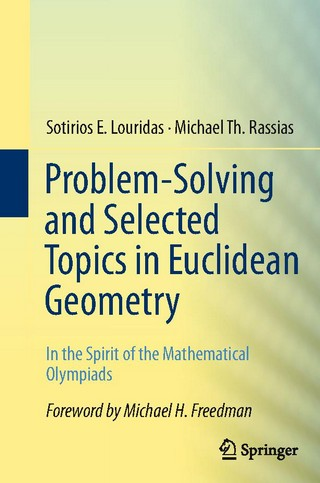### Book Toankho

Sách hay - Sách đẹp

# Problem-Solving and Selected Topics in Euclidean Geometry (In the Spirit of the Mathematical Olympiads)“Problem-Solving and Selected Topics in Euclidean Geometry: in the Spirit of the Mathematical Olympiads” contains theorems which are of particular value for the solution of geometrical problems. Emphasis is given in the discussion of a variety of methods, which play a significant role for the solution of problems in Euclidean Geometry. Before the complete solution of every problem, a key idea is presented so that the reader will be able to provide the solution. Applications of the basic geometrical methods which include analysis, synthesis, construction and proof are given. Selected problems which have been given in mathematical olympiads or proposed in short lists in IMO’s are discussed. In addition, a number of problems proposed by leading mathematicians in the subject are included here. The book also contains new problems with their solutions. The scope of the publication of the present book is to teach mathematical thinking through Geometry and to provide inspiration for both students and teachers to formulate “positive” conjectures and provide solutions.
Download ToanKho:
http://lk.libvui.com/Umgv
http://megaurl.io/XDHzT

loading...
Loading...